# Design and Analyze Compact UWB Low Pass Filter Using pcbComponent

This example shows how to design and analyze a compact low pass ultra-wide band (UWB) filter based on a U-shaped complementary split ring resonator using the pcbComponent object. The filter is designed to have very low insertion loss over a wide band of frequency from low 0.1 GHz to 10.8 GHz. The design of the filter is taken from the reference .

### Design and Analyze UWB Low Pass Filter

This compact filter design employs U-shaped complementary split ring resonator (U-CSRR). U-CSRR is a uniplanar configuration of complementary split ring resonator (CSRR) as described in . This structure has an advantage of simpler fabrication as it is formed on the top metal layer. The U-CSRR is formed using two concentric split square rings of outer length ${L}_{1}$, inner length ${L}_{2}$ as shown in Figure (a) below. This U-CSRR element is created on top metal layer of hosting transmission line. The equivalent circuit of the single U-CSRR cell shown in Figure (b) is represented by a parallel resonant circuit with inductance ${L}_{c}$ and capacitance ${C}_{c}$. The inductance ${L}_{R}$ and capacitance ${C}_{R}$ represent the inductance and capacitance of the host transmission. The U-CSRR particle is electrically coupled to the host transmission line.

The equivalent circuit of the U-CSRR particle suggests that at low frequency, the impedance of the parallel tank circuit is small and the circuit has passband characteristics. Figure (a) shows the schematic diagram of such a filter employing two U-CSRR cells in microstrip  representing various feature dimensions. As in , the choice of two U-CSRR particles in filter design is to have a compact size and ensure high attenuation in stop band.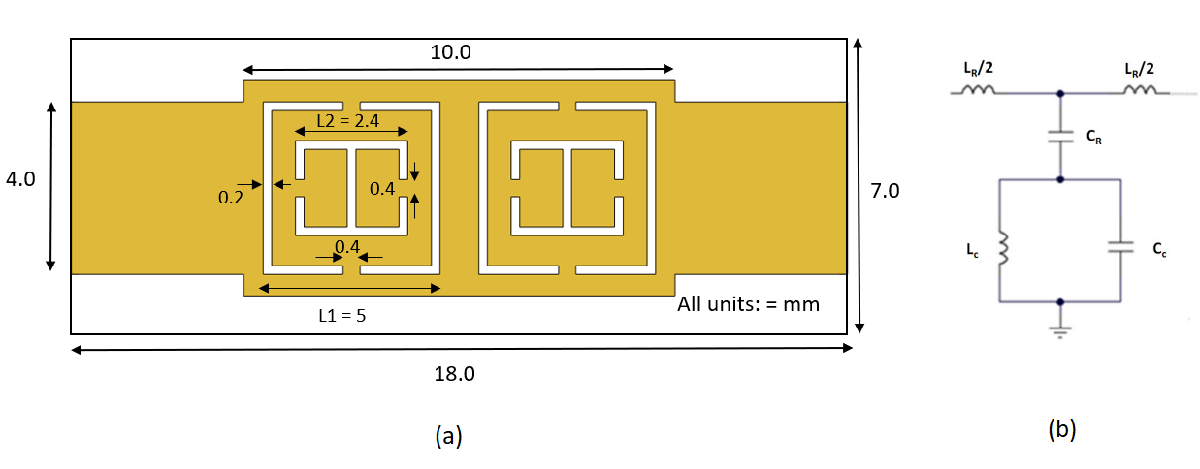Use the `traceRectangular` object to create feeding transmission line `ZA `and rectangular unit cell` Cell_A.` Perform the Boolean `add` operation for the microstrip shapes `ZA`, `Cell_A `and create `LeftSection`.

```% Set variables for ground plane gndL = 18e-3; gndW = 7e-3; % Set variables for feeding transmission line ZA_Width = 4e-3; ZA_Length = 4e-3; % Define unit cell length Cell_Length = 5e-3; % Create feeding microstrip line ZA = traceRectangular("Length",ZA_Length,"Width",ZA_Width,... "Center",[-ZA_Length/2-Cell_Length 0]); % Create rectangular unit cell Cell_A = traceRectangular("Length",Cell_Length,"Width",Cell_Length,... "Center",[-Cell_Length/2 0]); % Join feeding line and rectangular unit cell LeftSection = ZA + Cell_A;```

Use `traceLine` object to create shapes `s1, s2, s3, s4`. Use `traceRectangular` object to create shape `s5.`

Subtract shapes `s1,s2,s3,s4,` and `s5` from `LeftSection`. This operation creates various slots seen on U-CSRR particle. Visualize `LeftSection` using the `show`

```% Create shapes for various slots s1 = traceLine('StartPoint',[-Cell_Length/2-0.2e-3 -1.9e-3],... 'Angle',[-180 -270 0],'Length',[1.75e-3 3.8e-3 1.75e-3],'Width',0.2e-3); s2 = traceLine('StartPoint',[-Cell_Length/2+0.2e-3 -1.9e-3],... 'Angle',[0 90 180],'Length',[1.75e-3 3.8e-3 1.75e-3],'Width',0.2e-3); s3 = traceLine('StartPoint',[-Cell_Length/2-1.2e-3 -0.2e-3],... 'Angle',[-90 0 90],'Length',[0.8e-3 2.4e-3 0.8e-3],'Width',0.2e-3); s4 = traceLine('StartPoint',[-Cell_Length/2-1.2e-3 0.2e-3],... 'Angle',[90 0 -90],'Length',[0.8e-3 2.4e-3 0.8e-3],'Width',0.2e-3); s5 = traceRectangular("Length",0.2e-3,"Width",1.8e-3,... "Center",[-Cell_Length/2 0]); % Create slots of U-CSRR on hosted micrsotrip line LeftSection = LeftSection -s1 -s2 -s3 -s4 -s5; figure; show(LeftSection);```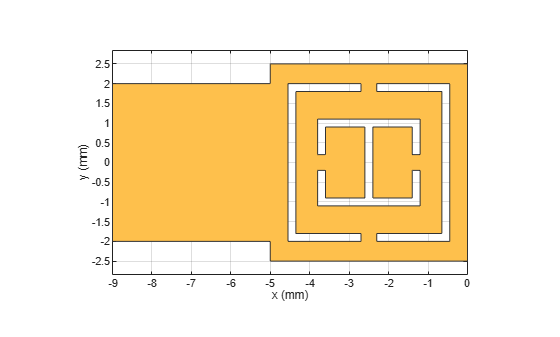Use the `copy`, `rotateZ,` and `rotateX` methods on the `LeftSection` object to create a `RightSection`. This creates right portion of filter having another U-CSRR hosted transmission line. Visualize `RightSection` using `show function.`

```RightSection = copy(LeftSection); RightSection = rotateZ(RightSection,180); RightSection = rotateX(RightSection,180); figure; show(RightSection);```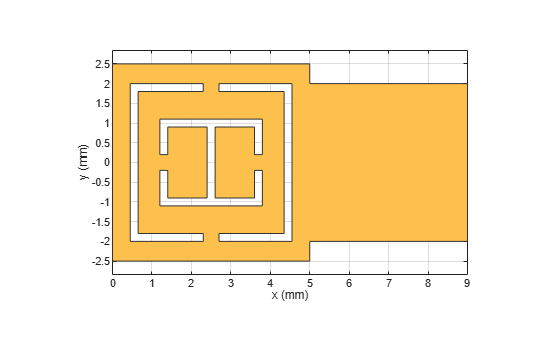Perform theBoolean `add` operation for the shapes `LeftSection`, `RightSection` to create a `filter`. Visualize the filter.

```filter = LeftSection + RightSection; show(filter);```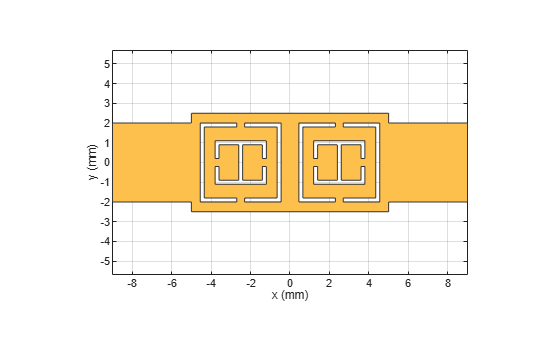Define the substrate parameters and create a dielectric to use in the `pcbComponent` of the designed filter. Create a groundplane using the `traceRectangular` shape. Use the `pcbComponent` to create a filter PCB. Assign the dielectric and ground plane to the `Layers` property on `pcbComponent`. Assign the `FeedLocations` to the edge of the feed ports. Set the `BoardThickness` to 1.52 mm on the `pcbComponent` and visualize the filter. The below code performs these operations and creates the filter PCB.

```% Define Substrate and its thickness substrate = dielectric("RO4730JXR"); substrate.Thickness = 1.52e-3; % Define bottom ground plane ground = traceRectangular("Length",gndL,"Width",gndW,... "Center",[0,0]); ```

Use pcbComponent to create a filter pcb

```pcb = pcbComponent; pcb.BoardShape = ground; pcb.BoardThickness = 1.52e-3; pcb.Layers ={filter,substrate,ground}; pcb.FeedDiameter = ZA_Width/2; pcb.FeedLocations = [-gndL/2 0 1 3;gndL/2 0 1 3]; figure; show(pcb);```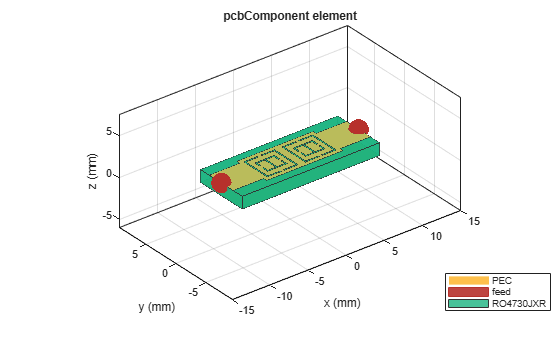Use the `mesh` function to have fine meshing and set `MaxEdgeLength to 1mm.`

```figure; mesh(pcb,'MaxEdgeLength',1e-3);```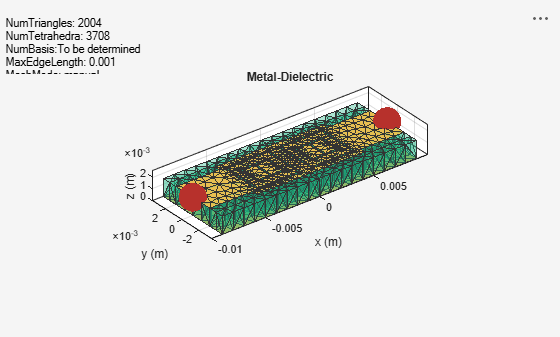Use the `sparameters` function to calculate the S-parameters for the low pass filter and plot it using the `rfplot` function.

```spar = sparameters(pcb,linspace(0.1e9,15e9,30)); figure; rfplot(spar);```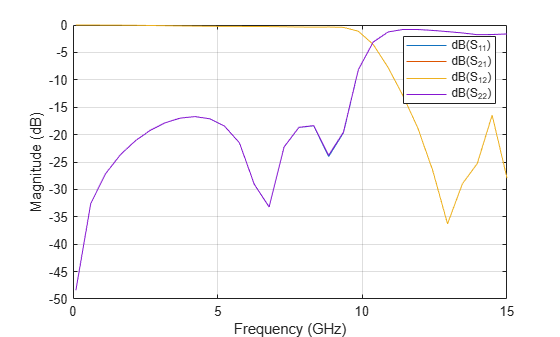As there are four curves in the result, let us analyze the results.

### Plot S-Parameters

Analyze the values of ${S}_{21}$, and ${S}_{11}$ to understand the behavior of low pass filter.

```figure; rfplot(spar,[1 2],1);```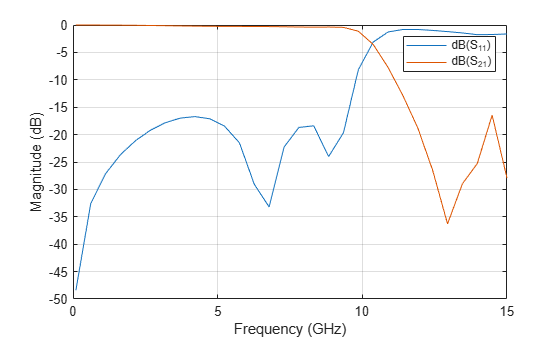The result shows that the filter has ${S}_{21}$ values close to 0 dB and ${S}_{11}$ values less than -15 dB between wide band of frequencies ${f}_{1}$ = 0.1 GHz and ${f}_{2}$ = 10.0 GHz. The designed filter therefore has ultra-wide passband response. For frequencies greater than 10.8 GHz, ${S}_{21}$ values are less than -10 dB indicating stopband response.

Use the `charge` function to visualize the charge distribution on the metal surface and dielectric of low pass filter.

```figure; charge(pcb,5e9);```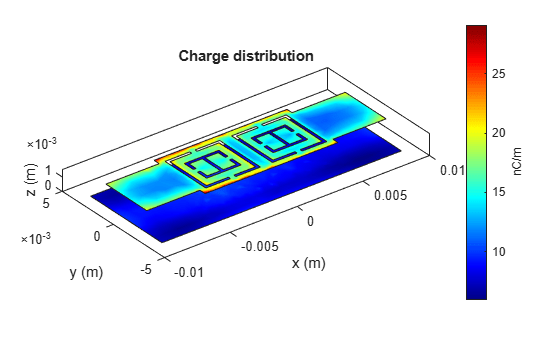```figure; charge(pcb,5e9,'dielectric');```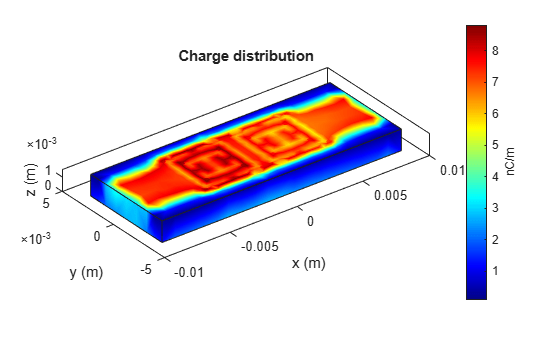Use the `current` function to visualize the current distribution on the metal surface and volume polarization currents on dielectric of low pass filter.

```figure; current(pcb,5e9);```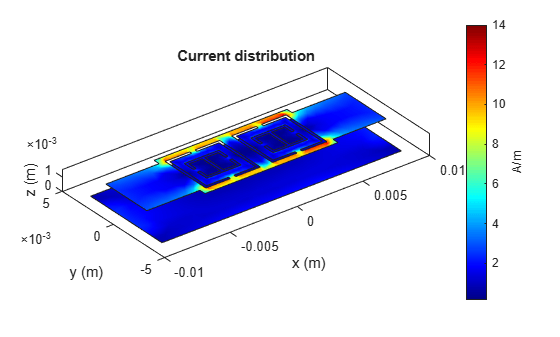```figure; current(pcb,5e9,'dielectric');```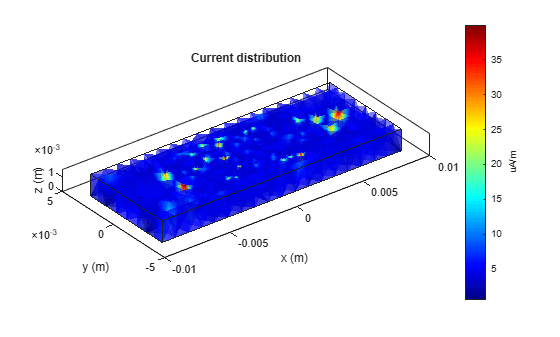### References

 Abdalla, M. A., G. Arafa, and M. Saad, “Compact UWB LPF based on uni-planar metamaterial complementary split ring resonator,” Proceedings of 10th International Congress on Advanced Electromagnetic Materials in Microwaves and Optics (METAMATERIALS), 10–12, Chania, Greece, Sep. 2016